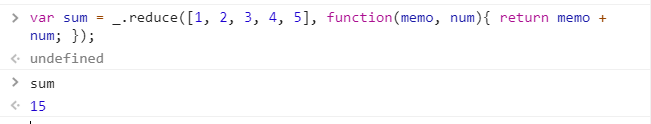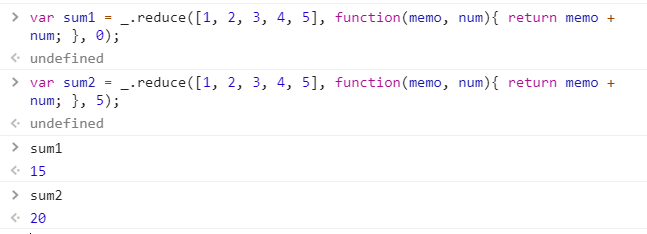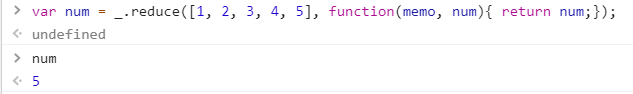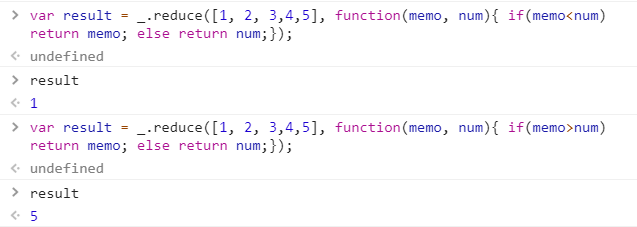# Underscore.js | _.reduce() Method

The _.reduce() method is an inbuilt method in Underscore.js which is used to transform an array’s/object’s properties into one single value or is used to create a single result from a given list of values. When all the elements of the list are passed to the function/iteratee and no more elements remains then the _.each loop ends.
The iteratee function makes use of memorization, i.e. it is remembering the return value each time it calculates a value.

Syntax:

`_.reduce(list, iteratee, memo)`

Parameters: This method accept three parameters as mentioned above and described below:

• list: It is the list containing some elements.
• iteratee: It is the function which is used to take all the elements of the list and it also remember all the returned value.
• memo: It is a value.

Return value: It returns the value of the last iteration is returned by the _.reduce() function.

JavaScript code to show the working of _.reduce() function

1) Passing a list of numbers to _.reduce() function: The ._reduce() function takes the element from the list one by one and do the specified operations on the code. Like here the operation is addition of the elements of the list. After adding all the elements, the reduce function ends. Here the starting value of memo is taken as ‘0’.

 `<``html``> ` `  `  `<``head``> ` `    ``<``script``> ` `        ``<``src` `= "https://cdnjs.cloudflare.com/ajax/libs/ ` `                ``underscore.js/1.9.1/underscore-min.js" > ` `    `` ` `    ``<``script` `type``=``"text/javascript"` `    ``src="https://cdnjs.cloudflare.com/ajax/libs/underscore.js ` `         ``/1.9.1/underscore-min.js.map"> ` `  `  `    ``<``script` `type``=``"text/javascript"` `     ``src="https://cdnjs.cloudflare.com/ajax/libs/underscore.js ` `          ``/1.9.1/underscore.js"> ` ` ` `  `  `<``body``> ` `    ``<``script` `type``=``"text/javascript"``> ` `          ``var sum=_.reduce([1, 2, 3, 4, 5], function(memo, num) { ` `               ``return memo + num;  ` `          ``}); ` `          ``document.write(sum);    ` `    `` ` ` ` `  `  ` `

Output:2) Passing and not passing the value of memo: If we are not passing memo variable’s value then it takes the value of the first element from the list. Otherwise it takes the mentioned value.

 `<``html``> ` `  `  `<``head``> ` `    ``<``script``> ` `        ``<``src` `= "https://cdnjs.cloudflare.com/ajax/libs/ ` `                ``underscore.js/1.9.1/underscore-min.js" > ` `    `` ` `    ``<``script` `type``=``"text/javascript"` `    ``src="https://cdnjs.cloudflare.com/ajax/libs/underscore.js ` `         ``/1.9.1/underscore-min.js.map"> ` `  `  `    ``<``script` `type``=``"text/javascript"` `     ``src="https://cdnjs.cloudflare.com/ajax/libs/underscore.js ` `          ``/1.9.1/underscore.js"> ` ` ` `  `  `<``body``> ` `    ``<``script` `type``=``"text/javascript"``> ` `         ``var sum1 = _.reduce([1, 2, 3, 4, 5], function(memo, num){  ` `               ``return memo + num;   ` `         ``0 }); ` `         ``var sum2 = _.reduce([1, 2, 3, 4, 5], function(memo, num){  ` `               ``return memo + num;   ` `         ``5 }); ` `         ``document.write(sum1); ` `         ``document.write(sum); ` `    `` ` ` ` `  `  ` `

Output:3) Finding out the value of num variable: The ‘num’ variable is a variable which stores the values of the list elements. Therefore since we are returning the value at the end when the function gets over, so, this implies that the list is also over. Therefore, the last element of the list will be printed.

 `<``html``> ` `  `  `<``head``> ` `    ``<``script``> ` `        ``<``src` `= "https://cdnjs.cloudflare.com/ajax/libs/ ` `                ``underscore.js/1.9.1/underscore-min.js" > ` `    `` ` `    ``<``script` `type``=``"text/javascript"` `    ``src="https://cdnjs.cloudflare.com/ajax/libs/underscore.js ` `         ``/1.9.1/underscore-min.js.map"> ` `  `  `    ``<``script` `type``=``"text/javascript"` `     ``src="https://cdnjs.cloudflare.com/ajax/libs/underscore.js ` `          ``/1.9.1/underscore.js"> ` ` ` `  `  `<``body``> ` `    ``<``script` `type``=``"text/javascript"``> ` `         ``var num=_.reduce([1, 2, 3, 4, 5], function(memo, num) { ` `              ``return num; ` `          ``}); ` `         ``document.write(num); ` `    `` ` ` ` `  `  ` `

Output:4) Applying logical operators in the _.reduce() function: From the above examples we are clear that in the value of memo is 1 and that of num is 5 (for this example only). So, we can apply the logical operator (>, <) to compare the values of num and memo and then print their values.

 `<``html``> ` `  `  `<``head``> ` `    ``<``script``> ` `        ``<``src` `= "https://cdnjs.cloudflare.com/ajax/libs/ ` `                ``underscore.js/1.9.1/underscore-min.js" > ` `    `` ` `    ``<``script` `type``=``"text/javascript"` `    ``src="https://cdnjs.cloudflare.com/ajax/libs/underscore.js ` `         ``/1.9.1/underscore-min.js.map"> ` `  `  `    ``<``script` `type``=``"text/javascript"` `     ``src="https://cdnjs.cloudflare.com/ajax/libs/underscore.js ` `          ``/1.9.1/underscore.js"> ` ` ` `  `  `<``body``> ` `    ``<``script` `type``=``"text/javascript"``> ` `         ``var result=_.reduce([1, 2, 3, 4, 5], function(memo, num) { ` `               ``if(memo<``num``) ` `                    ``return memo; ` `               ``else ` `                    ``return num; ` `         ``}); ` `    `` ` ` ` `  `  ` `

Output:My Personal Notes arrow_drop_upI like to do coding in C++C and java programming languages HTML and CSS always intersts me Sharing knowleged is the best way according to me to increase ones knwoledge

If you like GeeksforGeeks and would like to contribute, you can also write an article using contribute.geeksforgeeks.org or mail your article to contribute@geeksforgeeks.org. See your article appearing on the GeeksforGeeks main page and help other Geeks.

Please Improve this article if you find anything incorrect by clicking on the "Improve Article" button below.